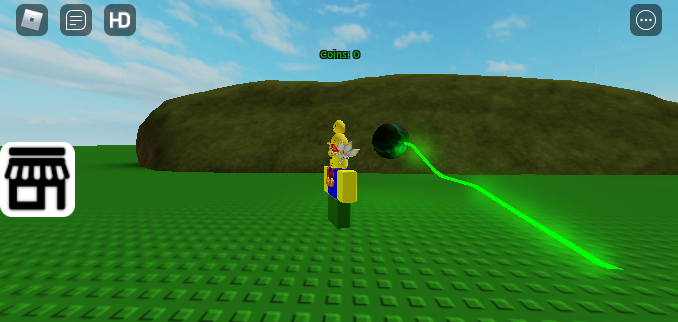# The parts and the sound don't get destroyed, still visible to players!?

You can write your topic however you want, but you need to answer these questions:

1. What do you want to achieve? Make the parts, the “Handle” and the sound get destroyed if the player die.

2. What is the issue? If the player die while the script is running, the parts & Handle don’t disappear and the sound keep playing.

3. What solutions have you tried so far? I’ve tried to do make different things but I still not figured out, I also tried to find a solution on Dev Forum but seems like there isn’t anything that can help me.

I’m just started learning script recently so I’m quite bad. This is a script inside a Roblox gear.
Here’s the script:
function MakeLightning(ray,atime)
local startTime = tick()
while tick()-startTime<atime do
local points = {}
for i=0,10,1 do
if i==0 then
table.insert(points,ray.Origin)
else
table.insert(points,ray.Origin+(ray.Direction.Unit*(ray.Direction.magnitude/10)*i)+(Vector3.new(math.random(),math.random(),math.random())*2))
end
end
local parts = {}
for index,i in pairs(points) do
if index >1 then
local npart = Instance.new(‘Part’)
npart.CanCollide = false
npart.FormFactor=‘Custom’
npart.Size= Vector3.new(.2,.2,(points[index]-points[index-1]).magnitude)
npart.Archivable = false
npart.Anchored = true
npart.CFrame = CFrame.new((points[index]+points[index-1])/2,points[index])
npart.Transparency=0.1
npart.BrickColor = BrickColor.new(‘Lime green’)
npart.Material = ‘Neon’
npart.Parent = game.Workspace

``````			npart.Touched:connect(function(npart)
local humanOther = npart.Parent:FindFirstChild("Humanoid")
local tool = script.Parent
if not humanOther then return end
if humanOther.Parent == tool then return end
if game.Players:GetPlayerFromCharacter(npart.Parent) then return end
if npart.Parent:FindFirstChild('Humanoid') then
npart.Parent.Humanoid:TakeDamage(250)
end
end)

table.insert(parts,npart)
end
end
wait(0.5)
for _,i in pairs(parts) do
i.Parent = nil
end
end
``````

end

local cframeValue = script:WaitForChild(‘cframe’)

local Handle = Instance.new(“Part”)
Handle.Anchored = true
Handle.Transparency = 0
Handle.TopSurface = Enum.SurfaceType.Smooth
Handle.Name = “Handle”
Handle.Size = Vector3.new(1, 1, 1.2)
Handle.BottomSurface = Enum.SurfaceType.Smooth
Handle.Locked = true
Handle.CanCollide = false
Handle.Archivable = false

``````local Mesh = Instance.new("SpecialMesh")
Mesh.Scale = Vector3.new(5, 5, 5)
Mesh.MeshId = "http://www.roblox.com/asset/?id=82326541"
Mesh.TextureId = "http://www.roblox.com/asset/?id=82327419"
Mesh.Parent = Handle
Mesh.MeshType = Enum.MeshType.FileMesh

local Sound = Instance.new('Sound')
Sound.Parent = Handle
Sound.SoundId = "http://www.roblox.com/asset/?id=127410465"
Sound.Volume = 0.1
Sound.Looped = true

local Light = Instance.new('PointLight')
Light.Color = Color3.new(128/255,255/255,49/255)
Light.Parent = Handle
``````

Handle.Parent = game.Workspace
Handle.CFrame=cframeValue.Value
Sound:Play()

MakeLightning(Ray.new(cframeValue.Value.p,cframeValue.Value.lookVector*(15+(math.random()*10))) ,6+(math.random()*2))

Sound:Destroy()
Handle:Destroy()
cframeValue.Parent = nil
cframeValue:Destroy()Parts and Handle still visible and sound keep playing.

Can you post the script? I may be able to help.

Here it is! Idk how to fix this

No one helped me to do this…

So, first of all check if it’s a LocalScript. I suppose it is not one because you seem like an experienced scripter.

The sound does not disappear because you set the “Lopped” bool to true. This means that it’ll go on forever even if the player dies. Though if the sound gets deleted, it’ll stop playing.

First of all, if the tool is in the StarterPack then you can just do “i:Destroy()”, this would destroy the parts instead of setting the Parent to nil. What you could also do is “game.Debris:AddItem(i)”, this would save some data usage.

Hope this helped!
SwedishRaptor